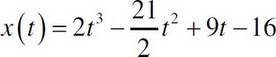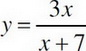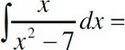# AP Calculus AB Practice Test 20

### Test Information7 questions21 minutes

Calculator Allowed

1. When is the particle whose path is described by, from t > 0, slowing down?

2. What is the area enclosed by the curve f(x) = 4x2 - x4 and the x-axis?

3. Which of the following is an asymptote for the curve?

4.5. What is the area between the curves y = x3 - 2x2 - 5x + 6 and y = x2 - x - 6 from x = -2 to x = 3 ?

6. Find the average value of f(x) = (3x - 1)3 on the interval from x = -1 to x = 3.

7. The curve y = ax2 + bx + c passes through the point (1, 5) and is normal to the line -x + 5y = 15 at (0, 3). What is the equation of the curve?